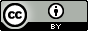# Schultz, T.W.; Netzeva, T.I.; Cronin, M.T.D. Selection of data sets for qsars: analyses of tetrahymena toxicity from aromatic compounds. SAR QSAR Environ. Res. 2003, 14, 1, 59–81.

## QsarDB content

### Property pIGC50: 40-h Tetrahymena toxicity as log(1/IGC50) [log(L/mmol)]

##### 2: All compounds

Regression model (regression)

Open in:QDB Explorer QDB Predictor

Name Type n

R2

σ

Training training 385 0.860 0.273
##### 3: Training compounds (n = 10)

Regression model (regression)

Open in:QDB Explorer QDB Predictor

Name Type n

R2

σ

Training training 10 0.977 0.215
##### 4: Training compounds (n = 15)

Regression model (regression)

Open in:QDB Explorer QDB Predictor

Name Type n

R2

σ

Training training 15 0.960 0.240
##### 5: Training compounds (n = 20)

Regression model (regression)

Open in:QDB Explorer QDB Predictor

Name Type n

R2

σ

Training training 20 0.957 0.235
##### 6: Training compounds (n = 25)

Regression model (regression)

Open in:QDB Explorer QDB Predictor

Name Type n

R2

σ

Training training 25 0.945 0.248
##### 7: Training compounds (n = 30)

Regression model (regression)

Open in:QDB Explorer QDB Predictor

Name Type n

R2

σ

Training training 30 0.927 0.302
##### 8: Training compounds (n = 35)

Regression model (regression)

Open in:QDB Explorer QDB Predictor

Name Type n

R2

σ

Training training 35 0.920 0.293
##### 9: Training compounds (n = 40)

Regression model (regression)

Open in:QDB Explorer QDB Predictor

Name Type n

R2

σ

Training training 40 0.913 0.297
##### 10: Training compounds (n = 45)

Regression model (regression)

Open in:QDB Explorer QDB Predictor

Name Type n

R2

σ

Training training 45 0.914 0.287
##### 11: Training compounds (n = 50)

Regression model (regression)

Open in:QDB Explorer QDB Predictor

Name Type n

R2

σ

Training training 50 0.911 0.292

## Citing

When using this QDB archive, please cite (see details) it together with the original article:

• Ruusmann, V. Data for: Selection of data sets for qsars: analyses of tetrahymena toxicity from aromatic compounds. QsarDB repository, QDB.66. 2012. http://dx.doi.org/10.15152/QDB.66

• Schultz, T. W.; Netzeva, T. I.; Cronin, M. T. D. Selection of data sets for qsars: analyses of tetrahymena toxicity from aromatic compounds. SAR QSAR Environ. Res. 2003, 14, 59–81. http://dx.doi.org/10.1080/1062936021000058782

 Title: Schultz, T.W.; Netzeva, T.I.; Cronin, M.T.D. Selection of data sets for qsars: analyses of tetrahymena toxicity from aromatic compounds. SAR QSAR Environ. Res. 2003, 14, 1, 59–81. Abstract: The aim of this investigation was to develop a strategy for the formulation of a valid ecotoxicological-based QSAR while, at the same time, minimizing the required number of toxicological data points. Two chemical selection approaches-distance-based optimality and K Nearest Neighbor (KNN), were used to examine the impact of the number of compounds used in the training and testing phases of QSAR development (i.e. diversity and representivity, respectively) on the predictivity (i.e. external validation) of the QSAR. Regression-based QSARs for the ectotoxic potency for population growth impairment of aromatic compounds (benzenes) to the aquatic ciliate Tetrahymena pyriformis were developed based on descriptors for chemical hydrophobicity and electrophilicity. A ratio of one compound in the training set to three in the test set was applied. The results indicate that from a known chemical universe, in this case 385 derivatives, robust QSARs of equal quality may be developed from a small number of diverse compounds, validated by a representative test set. As a conservative recommendation it is suggested that there should be a minimum of 10 observations for each variable in a QSAR. URI: http://hdl.handle.net/10967/66 http://dx.doi.org/10.15152/QDB.66 Date: 2012-05-23
﻿

## Files in this item

Name Description Format Size View
713745890.qdb.zip n/a application/zip 22.97Kb View/OpenFiles associated with this item are distributed